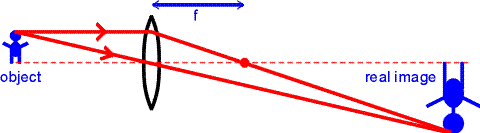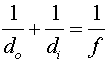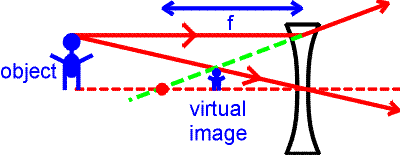Images, real and virtual

Real images are those where light actually converges, whereas virtual images are locations from where light appears to have converged. Real images occur when objects are placed outside the focal length of a converging lens or outside the focal length of a converging mirror. A real image is illustrated below. Ray tracing gives the position of the images by drawing one ray perpendicular to the lens, which must pass through the focal point, and a second ray that passes through the center of the lens, which is not bent by the lens. The intersection of the two rays gives the position of the image. (A third ray could be drawn which passes through the focal point on the left side of the lens; after passing through the lens, it would travel parallel to the axis, and would intersect the other two rays at the point where those rays already intersect. Note that the real image is inverted. (The image happens to be larger than the object. That happens because the object is between f and 2f away from the lens; if the lens were farther away than 2f, the image would be closer to the lens than 2f, and would be smaller than the object.)The position of the image can be found through the equation:Here, the distances are those of the object and image respectively as measured from the lens. The focal length f is positive for a convex lens. A positive image distance corresponds to a real image, just as it did for the case of the mirrors. However, for a lens, a positive image distance implies that the image is located on the opposite side from the object. (You will have no trouble remembering this if you think about it in the right way: a real image has to be where the light is, which means in front of a mirror, or behind a lens.)

Virtual images are formed by diverging lenses or by placing an object inside the focal length of a converging lens. The ray-tracing exercise is repeated for the case of a virtual image.In this case the virtual image is upright and shrunken. The same formula for the image and object distances used above applies again here. Only in this case the focal length is negative, and the solution for the image distance will also be negative. Virtual images can also be produced by converging lenses when the object is placed inside the focal length. In that case, the virtual image will be upright and enlarged, as it will be further from the lens than the object.

Lenses index      examples        Lecture index